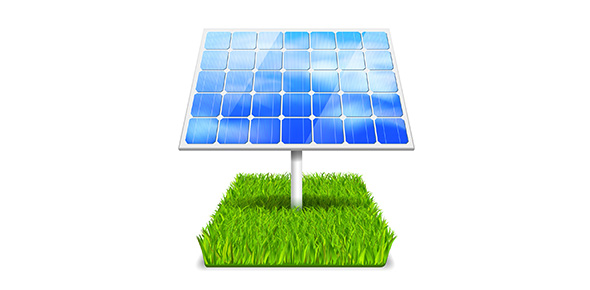# Energy Unit Practice Quiz Questions

12 Questions | Attempts: 108
ShareSettings• 1.
What is the Law of Conservation of Energy?
• A.

A law that states that energy cannot be created nor destroyed, but can change forms

• B.

A law stating that energy must be conserved in order to delay the apocalypse (the end of the world)

• C.

A trip to Disney World

• D.

The key to the house

• 2.
What is potential energy?
• A.

Energy (stored) due to position or condition

• B.

The key to the meaning of life

• C.

Sarah's high school diploma

• D.

None of the above

• 3.
Releasing a compressed spring is not an example of kinetic energy being converted to potential energy
• A.

True

• B.

False

• 4.
At the top of its arc, a thrown ball has _____________ potential energy
• 5.
The brakes on a car exert a frictional force of 6000 N to stop the car. If the work done by the brakes is 120,000 J to stop the car, how many meters did the car travel before it stopped?
• A.

20 m

• B.

6 m

• C.

72 m

• D.

12 m

• 6.
A man pushed a crate along a floor by exerting a force of 55 N. If the crate moves 4.0 m, how much work does the man do?
• A.

165 J

• B.

220 J

• C.

Zero

• D.

200J

• 7.
Which of the following statements about work and energy is NOT true?
• A.

When work is done, energy is transferred or transformed

• B.

Work and energy are always equal

• C.

• D.

Work and energy have the same units

• 8.
How are energy and work related?
• A.

Energy = the ability to do work

• B.

Work = transfer of energy (moves an object)

• C.

BOTH are measured in Joules

• D.

All of the above

• 9.
A boy pushes on a parked car with a force of 200N. The car does not move. How much work does the boy do?
• A.

200N

• B.

720N

• C.

Zero

• D.

None of the above

• 10.
Which form of energy does a plant store when light is transformed during photosynthesis
• A.

Chemical

• B.

Thermal

• C.

Mechanical

• D.

Electrical

• 11.
Friction causes kinetic energy to be turned into ____________.
• A.

Mechanical energy

• B.

Solar energy

• C.

Thermal energy

• D.

All of the above

• 12.
Which of the following is NOT an example of kinetic energy being converted to potential energy?
• A.

• B.

Releasing a compressed spring

• C.

Pulling a sled up a hill

• D.

None of the above

## Related TopicsBack to top
×

Wait!
Here's an interesting quiz for you.